Miscellaneous

Chapter 4 Class 12 Determinants
Serial order wise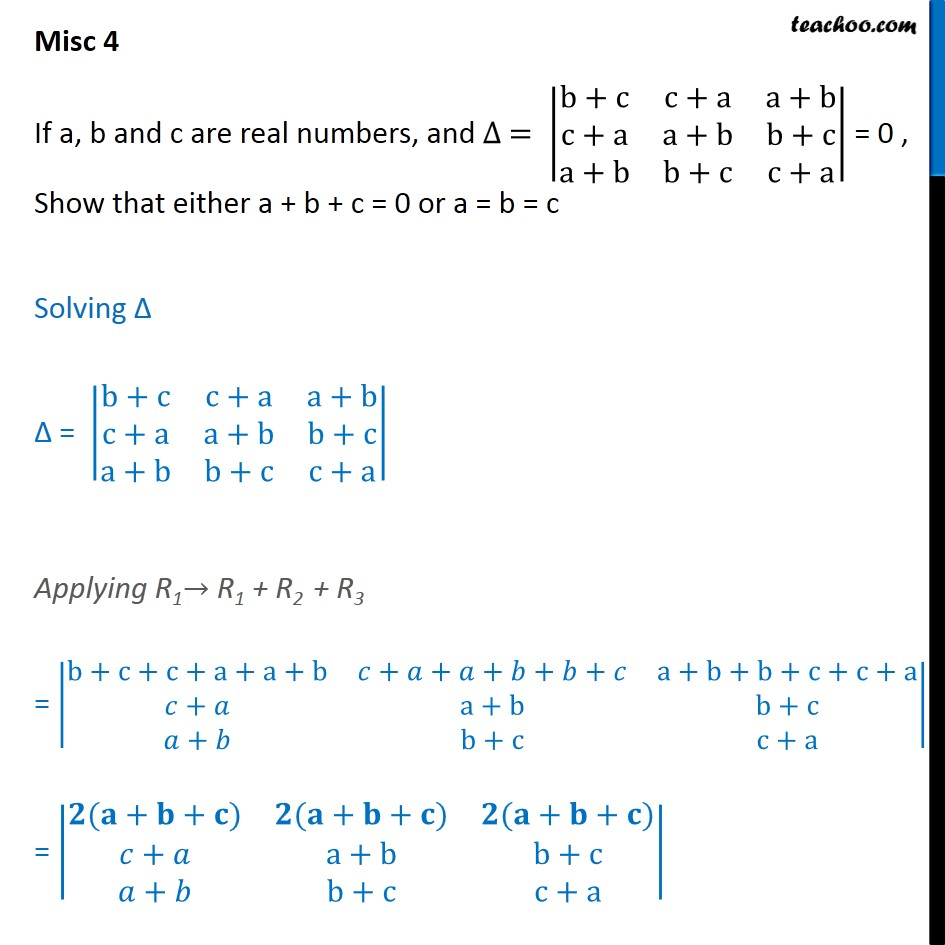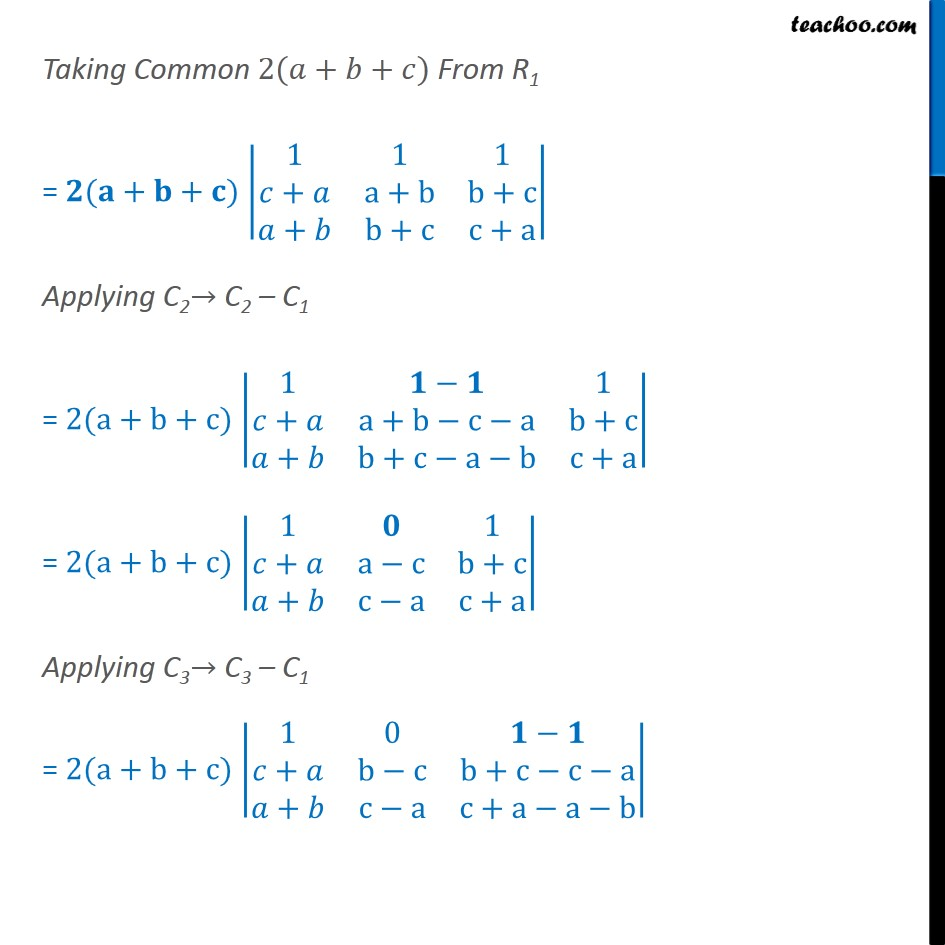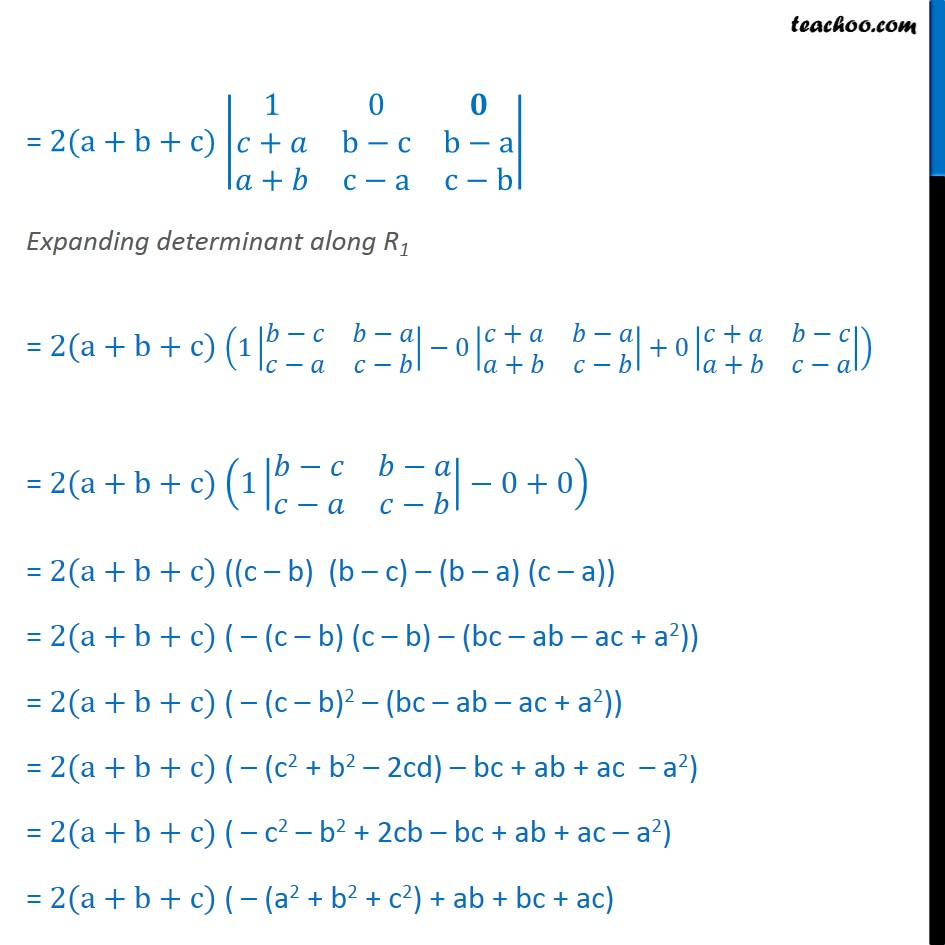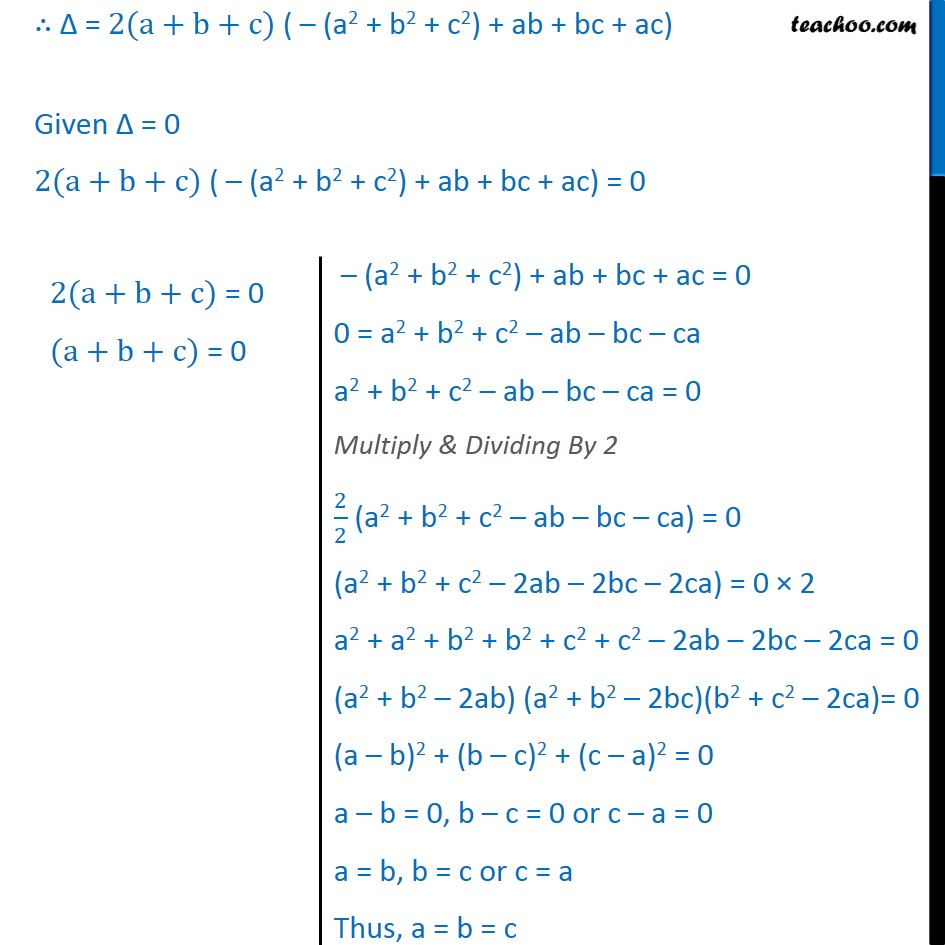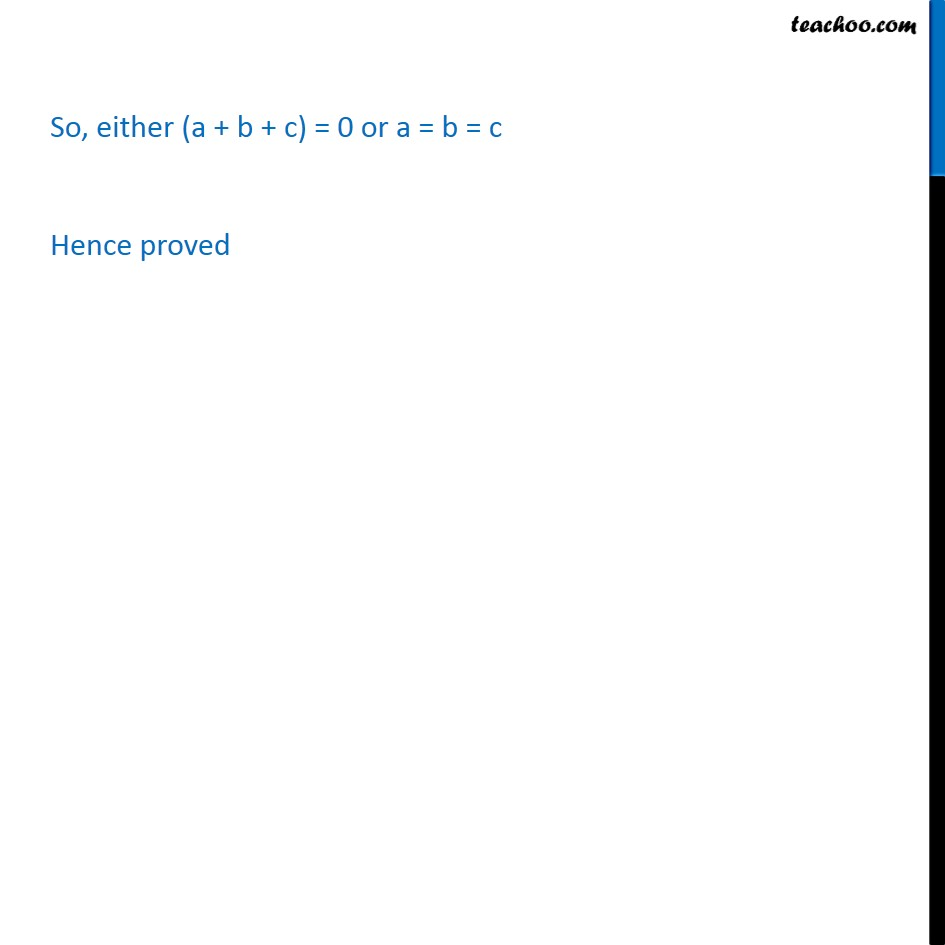Learn in your speed, with individual attention - Teachoo Maths 1-on-1 Class

### Transcript

Question 2 If a, b and c are real numbers, and ∆ = b+c﷮c+a﷮a+b﷮c+a﷮a+b﷮b+c﷮a+b﷮b+c﷮c+a﷯﷯ = 0 , Show that either a + b + c = 0 or a = b = c Solving ∆ ∆ = b+c﷮c+a﷮a+b﷮c+a﷮a+b﷮b+c﷮a+b﷮b+c﷮c+a﷯﷯ Applying R1→ R1 + R2 + R3 = b+c+c+a+a+b﷮𝑐+𝑎+𝑎+𝑏+𝑏+𝑐﷮a+b+b+c+c+a﷮𝑐+𝑎﷮a+b﷮b+c﷮𝑎+𝑏﷮b+c﷮c+a﷯﷯ = 𝟐(𝐚+𝐛+𝐜)﷮𝟐(𝐚+𝐛+𝐜)﷮𝟐(𝐚+𝐛+𝐜)﷮𝑐+𝑎﷮a+b﷮b+c﷮𝑎+𝑏﷮b+c﷮c+a﷯﷯ Taking Common 2(𝑎+𝑏+𝑐) From R1 = 𝟐(𝐚+𝐛+𝐜) 1﷮1﷮1﷮𝑐+𝑎﷮a+b﷮b+c﷮𝑎+𝑏﷮b+c﷮c+a﷯﷯ Applying C2→ C2 – C1 = 2(a+b+c) 1﷮𝟏−𝟏﷮1﷮𝑐+𝑎﷮a+b−c−a﷮b+c﷮𝑎+𝑏﷮b+c−a−b﷮c+a﷯﷯ = 2(a+b+c) 1﷮𝟎﷮1﷮𝑐+𝑎﷮a−c﷮b+c﷮𝑎+𝑏﷮c−a﷮c+a﷯﷯ Applying C3→ C3 – C1 = 2(a+b+c) 1﷮0﷮𝟏−𝟏﷮𝑐+𝑎﷮b−c﷮b+c−c−a﷮𝑎+𝑏﷮c−a﷮c+a−a−b﷯﷯ = 2(a+b+c) 1﷮0﷮𝟎﷮𝑐+𝑎﷮b−c﷮b−a﷮𝑎+𝑏﷮c−a﷮c−b﷯﷯ Expanding determinant along R1 = 2(a+b+c) 1 𝑏−𝑐﷮𝑏−𝑎﷮𝑐−𝑎﷮𝑐−𝑏﷯﷯−0 𝑐+𝑎﷮𝑏−𝑎﷮𝑎+𝑏﷮𝑐−𝑏﷯﷯+0 𝑐+𝑎﷮𝑏−𝑐﷮𝑎+𝑏﷮𝑐−𝑎﷯﷯﷯ = 2(a+b+c) 1 𝑏−𝑐﷮𝑏−𝑎﷮𝑐−𝑎﷮𝑐−𝑏﷯﷯−0+0﷯ = 2(a+b+c) ((c – b) (b – c) – (b – a) (c – a)) = 2(a+b+c) ( – (c – b) (c – b) – (bc – ab – ac + a2)) = 2(a+b+c) ( – (c – b)2 – (bc – ab – ac + a2)) = 2(a+b+c) ( – (c2 + b2 – 2cd) – bc + ab + ac – a2) = 2(a+b+c) ( – c2 – b2 + 2cb – bc + ab + ac – a2) = 2(a+b+c) ( – (a2 + b2 + c2) + ab + bc + ac) ∴ ∆ = 2(a+b+c) ( – (a2 + b2 + c2) + ab + bc + ac) Given ∆ = 0 2(a+b+c) ( – (a2 + b2 + c2) + ab + bc + ac) = 0 So, either (a + b + c) = 0 or a = b = c Hence proved